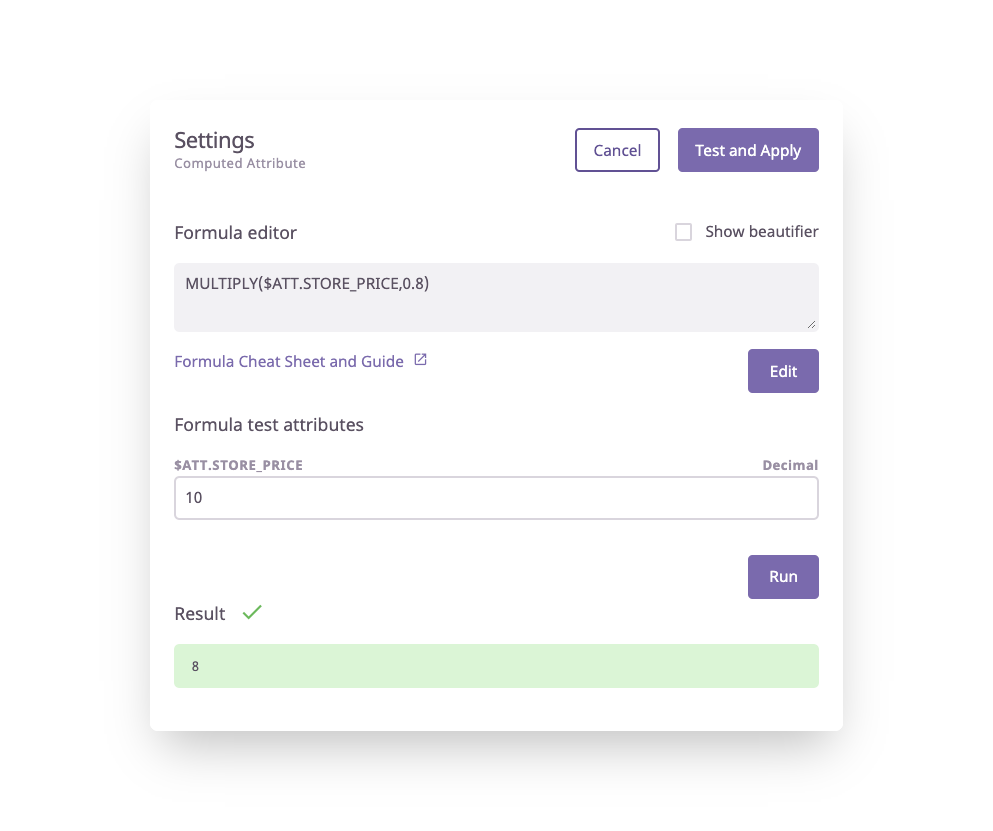# MULTIPLY Operation

## How to use the MULTIPLY operation

Definition

Example

Syntax Guide

Formula In Use

### Definition

The MULTIPLY operation returns the product of two or more numbers.

### Example

MULTIPLY(1, 2, 3, 4)

Result:
24

### Syntax Guide

MULTIPLY(value1,value2,[value3…])

value1 - The first multiplicand

value2 - The second multiplicand

[value3..]. - [OPTIONAL] additional values to multiply

### Formula In Use

MULTIPLY(\$ATT.STORE_PRICE,0.8)

In this example, we are applying a discount of 20% to the store price attribute.### What's Next?

If you have any questions just click on the chat box in the bottom-right corner and we'll be happy to answer them...

and please let us know 👇